kacey@ieee.org

# Reinforcement Learning - Policy Gradient Methods¶

Objective:

• Use open source reinforcement learning (RL) environments
• Set up the training pipelines for RL
• Testing different environments and reward engineering
• Implementing Policy Gradient method REINFORCE and its variation with a baseline function.
In [ ]:


In :
import numpy as np
import matplotlib.pyplot as plt

%matplotlib inline
plt.rcParams['figure.figsize'] = (10.0, 8.0) # set default size of plots
plt.rcParams['image.interpolation'] = 'nearest'
plt.rcParams['image.cmap'] = 'gray'


In [ ]:
# SETUP

#install gym-minigrid:  https://github.com/maximecb/gym-minigrid


## Part 1: Getting familiar with gym-minigrid¶

We will be working with the gym-minigrid package, an open source, efficient implementation of a 2D gridworld environment for quickly training policies and verifying Policy Gradients.

You can find the package documentation here: https://github.com/maximecb/gym-minigrid

### Rendering images from the environment¶

To debug the progress of our RL loop we will need to render the state of the environment. Here we show how to load an instance of the environment type MiniGrid-Empty-5x5-v0, reset it, selecting an action, taking an action step in the environment, and finally rendering the current state.

In :
import gym
import gym_minigrid
import matplotlib.pyplot as plt

# load, reset, and render an instance of the environment ('MiniGrid-Empty-5x5-v0')
env = gym.make('MiniGrid-Empty-5x5-v0')
env.reset()
before_img = env.render('rgb_array')

# take an action and render the resulting state
action = env.actions.forward
obs, reward, done, info = env.step(action)
after_img = env.render('rgb_array')

plt.imshow(np.concatenate([before_img, after_img], 1))

Out:
<matplotlib.image.AxesImage at 0x7fc138cf9320>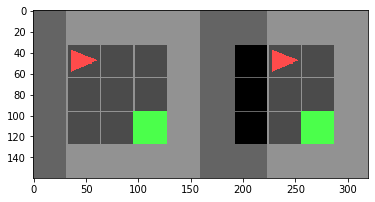Following the above code, Create an instance of the environment type MiniGrid-Empty-8x8-v0, reset, take an action step right and get the outputs of the environment's step() function. Finally, render the new state of the environment to visualize it.

In :
# 1. Make a new environment MiniGrid-Empty-8x8-v0
env = gym.make('MiniGrid-Empty-8x8-v0')

# 2. Reset the environment
env.reset()
# before_img = env.render('rgb_array')

# 3. Select the action right
action = env.actions.right

# 4. Take a step in the environment and store it in appropriate variables
obs, reward, done, info = env.step(action)

# 5. Render the current state of the environment
img = env.render('rgb_array')
# plt.imshow(np.concatenate([before_img, img], 1))

print('Observation:', obs)
print('Reward:', reward)
print('Done:', done)
print('Info:', info)
print('Image shape:', img.shape)
plt.imshow(img)
plt.show()

Observation: {'image': array([[[2, 5, 0],
[1, 0, 0],
[1, 0, 0],
[1, 0, 0],
[1, 0, 0],
[1, 0, 0],
[1, 0, 0]],

[[2, 5, 0],
[1, 0, 0],
[1, 0, 0],
[1, 0, 0],
[1, 0, 0],
[1, 0, 0],
[1, 0, 0]],

[[2, 5, 0],
[1, 0, 0],
[1, 0, 0],
[1, 0, 0],
[1, 0, 0],
[1, 0, 0],
[1, 0, 0]],

[[2, 5, 0],
[1, 0, 0],
[1, 0, 0],
[1, 0, 0],
[1, 0, 0],
[1, 0, 0],
[1, 0, 0]],

[[2, 5, 0],
[2, 5, 0],
[2, 5, 0],
[2, 5, 0],
[2, 5, 0],
[2, 5, 0],
[2, 5, 0]],

[[2, 5, 0],
[2, 5, 0],
[2, 5, 0],
[2, 5, 0],
[2, 5, 0],
[2, 5, 0],
[2, 5, 0]],

[[2, 5, 0],
[2, 5, 0],
[2, 5, 0],
[2, 5, 0],
[2, 5, 0],
[2, 5, 0],
[2, 5, 0]]], dtype=uint8), 'direction': 1, 'mission': 'get to the green goal square'}
Reward: 0
Done: False
Info: {}
Image shape: (256, 256, 3)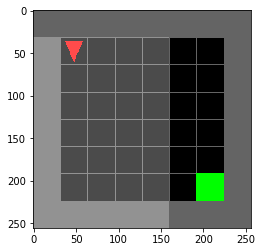## Modifying an existing environment¶

As you can see the environment's observation output also contains a rendered image as state representation (obs['image']). However, for this exercise we want to train out deep RL agent from non-image state input (for faster convergence).

We will create a slightly altered version of the FullyObsWrapper from the repository who's observation function returns a flat vector representing the full grid.

In :
import gym
from gym import spaces
from gym_minigrid.minigrid import OBJECT_TO_IDX, COLOR_TO_IDX

max_env_steps = 50

class FlatObsWrapper(gym.core.ObservationWrapper):
"""Fully observable gridworld returning a flat grid encoding."""

def __init__(self, env):
super().__init__(env)

# Since the outer walls are always present, we remove left, right, top, bottom walls
# from the observation space of the agent. There are 3 channels, but for simplicity,
# we will deal with flattened version of state.

self.observation_space = spaces.Box(
low=0,
high=255,
shape=((self.env.width-2) * (self.env.height-2) * 3,),  # number of cells
dtype='uint8'
)
self.unwrapped.max_steps = max_env_steps

def observation(self, obs):
# this method is called in the step() function to get the observation
# we provide code that gets the grid state and places the agent in it
env = self.unwrapped
full_grid = env.grid.encode()
full_grid[env.agent_pos][env.agent_pos] = np.array([
OBJECT_TO_IDX['agent'],
COLOR_TO_IDX['red'],
env.agent_dir
])
full_grid = full_grid[1:-1, 1:-1]   # remove outer walls of the environment (for efficiency)
flattened_grid = full_grid.flatten()

return flattened_grid

def render(self, *args, **kwargs):
"""This removes the default visualization of the partially observable field of view."""
kwargs['highlight'] = False
return self.unwrapped.render(*args, **kwargs)

env_name='MiniGrid-Empty-8x8-v0'
env=FlatObsWrapper(gym.make(env_name))

action = env.actions.right
obs, reward, done, info = env.step(action)
img = env.render('rgb_array')

print('Observation:', obs, ', Observation Shape: ', obs.shape)
print('Reward:', reward)
print('Done:', done)
print('Info:', info)
print('Image shape:', img.shape)
plt.close()
plt.imshow(img)
plt.show()

Observation: [10  0  1  1  0  0  1  0  0  1  0  0  1  0  0  1  0  0  1  0  0  1  0  0
1  0  0  1  0  0  1  0  0  1  0  0  1  0  0  1  0  0  1  0  0  1  0  0
1  0  0  1  0  0  1  0  0  1  0  0  1  0  0  1  0  0  1  0  0  1  0  0
1  0  0  1  0  0  1  0  0  1  0  0  1  0  0  1  0  0  1  0  0  1  0  0
1  0  0  1  0  0  1  0  0  8  1  0] , Observation Shape:  (108,)
Reward: 0
Done: False
Info: {}
Image shape: (256, 256, 3)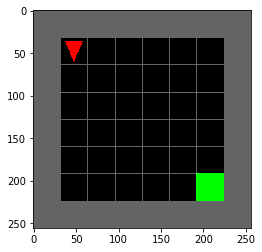### Plotting Videos¶

As final step in the environment setup, let's make sure we can see the logged video by testing the helper function show_video from utils.py

In :
from gym.wrappers import Monitor
from utils import show_video

# Monitor is a gym wrapper, which helps easy rendering of videos of the wrapped environment.
def wrap_env(env):
env = Monitor(env, './video', force=True)
return env

def gen_wrapped_env(env_name):
return wrap_env(FlatObsWrapper(gym.make(env_name)))

# Random agent - we only use it in this cell for demonstration
class RandPolicy:
def __init__(self, action_space):
self.action_space = action_space

def act(self, *unused_args):
return self.action_space.sample(), None

# This function plots videos of rollouts (episodes) of a given policy and environment
def log_policy_rollout(policy, env_name, pytorch_policy=False):
# Create environment with flat observation
env = gen_wrapped_env(env_name)

# Initialize environment
observation = env.reset()

done = False
episode_reward = 0
episode_length = 0

# Run until done == True
while not done:
# Take a step
if pytorch_policy:
observation = torch.tensor(observation, dtype=torch.float32)
action = policy.act(observation).data.cpu().numpy()
else:
action = policy.act(observation)
observation, reward, done, info = env.step(action)

episode_reward += reward
episode_length += 1

print('Total reward:', episode_reward)
print('Total length:', episode_length)

env.close()

show_video()

# Test that the logging function is working
test_env_name = 'MiniGrid-Empty-8x8-v0'
rand_policy = RandPolicy(FlatObsWrapper(gym.make(test_env_name)).action_space)
log_policy_rollout(rand_policy, test_env_name)

Total reward: 0
Total length: 50


## 2. Constructing the Rollout Buffer¶

Now that we can create an environment instance we can start implementing the components of the policy training framework. On a very high level, the policy gradient training loop looks like this:

1. Collect rollouts of the current policy, store all trajectories.
2. Compute policy gradient using stored rollouts. Update the policy.
3. Repeat.
In :
from torch.utils.data.sampler import BatchSampler, SubsetRandomSampler

class RolloutStorage():
def __init__(self, rollout_size, obs_size):
self.rollout_size = rollout_size
self.obs_size = obs_size
self.reset()

def insert(self, step, done, action, log_prob, reward, obs):
self.done[step].copy_(done)
self.actions[step].copy_(action)
self.log_probs[step].copy_(log_prob)
self.rewards[step].copy_(reward)
self.obs[step].copy_(obs)

def reset(self):
self.done = torch.zeros(self.rollout_size, 1)
self.actions = torch.zeros(self.rollout_size, 1, dtype=torch.int64)  # Assuming Discrete Action Space
self.log_probs = torch.zeros(self.rollout_size, 1)
self.rewards = torch.zeros(self.rollout_size, 1)
self.obs = torch.zeros(self.rollout_size, self.obs_size)

def compute_returns(self, gamma):
self.last_done = (self.done == 1).nonzero().max()  # Compute Returns until the last finished episode
self.returns[self.last_done+1] = 0.

# Accumulate discounted returns
for step in reversed(range(self.last_done+1)):
self.returns[step] = self.returns[step + 1] * \
gamma * (1 - self.done[step]) + self.rewards[step]

def batch_sampler(self, batch_size, get_old_log_probs=False):
sampler = BatchSampler(
SubsetRandomSampler(range(self.last_done)),
batch_size,
drop_last=True)
for indices in sampler:
if get_old_log_probs:
yield self.actions[indices], self.returns[indices], self.obs[indices], self.log_probs[indices]
else:
yield self.actions[indices], self.returns[indices], self.obs[indices]


## 3. Constructing the Policy Network¶

Now that we can store rollouts we need a policy to collect them. In the following you will complete the provided base code for the policy class. Using the pytorch modules nn.Sequential and nn.Linear, the policy is instantiated as a small neural network with simple fully-connected layers, the ActorNetwork.

After the network is constructed we can complete the Policy class that uses the actor to implement the act(...) and update(...) functions.

The gradient function for the policy update takes the following form:

$$\nabla J(\theta) = \mathbb{E}_{\pi}\big[ \nabla_{\theta} \log \pi_{\theta}(a, s) \; V_t(s) \big]$$

Here, $\theta$ are the parameters of the policy network $\pi_{\theta}$ and $V_t(s)$ is the observed future discounted reward from state $s$ onwards which should be maximized.

Entropy Loss: In order to encourage exploration, it is a common practice to add a weighted entropy-loss component that maximizes the entropy ($\mathcal{H}$) of the policy distribution, so it takes diverse actions. So the joint objective becomes:

$$\nabla J(\theta) = \mathbb{E}_{\pi}\big[ \nabla_{\theta} \log \pi_{\theta}(a, s) \; V_t(s) \big] + \nabla_{\theta}\mathcal{H}\big[\pi_\theta(a, s)\big]$$
In :
import torch
import torch.nn as nn
import torch.optim as optim
import torch.nn.functional as F
from torch.distributions.categorical import Categorical
from utils import count_model_params

class ActorNetwork(nn.Module):
def __init__(self, num_inputs, num_actions, hidden_dim):
super().__init__()
self.num_actions = num_actions
####################################################################################
### 1. Build the Actor network as a torch.nn.Sequential module                   ###
###    with the following layers:                                                ###
###        (1) a Linear layer mapping from input dimension to hidden dimension   ###
###        (2) a Tanh non-linearity                                              ###
###        (3) a Linear layer mapping from hidden dimension to hidden dimension  ###
###        (4) a Tanh non-linearity                                              ###
###        (5) a Linear layer mapping from hidden dimension to number of actions ###
### Note: We do not need an activation on the output, because the actor is       ###
###       predicting logits, not action probabilities.                           ###
####################################################################################
self.fc = nn.Sequential(
nn.Linear(num_inputs, hidden_dim),
nn.Tanh(),
nn.Linear(hidden_dim,hidden_dim),
nn.Tanh(),
nn.Linear(hidden_dim,num_actions),
nn.Softmax(dim=-1)
)

def forward(self, state):
x = self.fc(state)
return x

class Policy():
def __init__(self, num_inputs, num_actions, hidden_dim, learning_rate,
batch_size, policy_epochs, entropy_coef=0.001):
self.actor = ActorNetwork(num_inputs, num_actions, hidden_dim)
self.batch_size = batch_size
self.policy_epochs = policy_epochs
self.entropy_coef = entropy_coef

def act(self, state):
####################################################################################
### 1. Run the actor network on the current state to retrieve the action logits  ###
### 2. Build a Categorical(...) instance from the logits                         ###
### 3. Sample an action from the Categorical using the build-in sample() function###
####################################################################################

logits = self.actor.forward(state)
dist = Categorical(logits)
action = dist.sample()
log_prob = dist.log_prob(action)
return action, log_prob

def evaluate_actions(self, state, action):
####################################################################################
### This function is used for policy update to evaluate log_prob and entropy of  ###
### actor network.                                                               ###
####################################################################################
logits = self.actor.forward(state)
dist = Categorical(logits)
log_prob = dist.log_prob(action.squeeze(-1)).view(-1, 1)
entropy = dist.entropy().view(-1, 1)
return log_prob, entropy

def update(self, rollouts):
for epoch in range(self.policy_epochs):
data = rollouts.batch_sampler(self.batch_size)

for sample in data:
actions_batch, returns_batch, obs_batch = sample

log_probs_batch, entropy_batch = self.evaluate_actions(obs_batch, actions_batch)
####################################################################################
### 4. Compute the mean loss for the policy update using action log-             ###
###     probabilities and policy returns                                         ###
### 5. Compute the mean entropy for the policy update                            ###
###    *NOTE*: PyTorch optimizer is used to minimize by default.                 ###
###     The trick to maximize a quantity is to negate its corresponding loss.    ###
####################################################################################
policy_loss = torch.mean(torch.sum(torch.mul(log_probs_batch, returns_batch).mul(-1), -1))
entropy_loss = torch.mean(entropy_batch).mul(-1) #/self.batch_size

loss = policy_loss + self.entropy_coef * entropy_loss

loss.backward(retain_graph=False)
self.optimizer.step()

@property
def num_params(self):
return count_model_params(self.actor)


## 4. Setting up the Training Loop¶

Now that all classes are implemented we can combine them in the training loop and check whether everything is working!

Note about setting seeds: RL training is notoriously unstable. Especially in the beginning of training random chance can have a large influence on the training progress, which is why RL researchers always need to report results averaged across multiple random seeds. We therefore fix the random seed at the beginning of training. However, if you observe that your algorithm is not training it can be beneficial to try another run with a different random seed to check whether the last run was just "unlucky".

In :
from IPython.display import clear_output
from utils import AverageMeter, plot_learning_curve
import time

def train(env, rollouts, policy, params, seed=123):
# SETTING SEED: it is good practice to set seeds when running experiments to keep results comparable
np.random.seed(seed)
torch.manual_seed(seed)
env.seed(seed)

rollout_time, update_time = AverageMeter(), AverageMeter()  # Loggers
rewards, success_rate = [], []

print("Training model with {} parameters...".format(policy.num_params))

# Training Loop
## Initialization
avg_eps_reward, avg_success_rate = AverageMeter(), AverageMeter()
done = False
prev_obs = env.reset()
prev_obs = torch.tensor(prev_obs, dtype=torch.float32)
eps_reward = 0.
start_time = time.time()

## Collect rollouts
for step in range(rollouts.rollout_size):
if done:
# Store episode statistics
avg_eps_reward.update(eps_reward)
if 'success' in info:
avg_success_rate.update(int(info['success']))

# Reset Environment
obs = env.reset()
obs = torch.tensor(obs, dtype=torch.float32)
eps_reward = 0.
else:
obs = prev_obs

####################################################################################
### 1. Call the policy to get the action for the current observation,            ###
### 2. Take one step in the environment (using the policy's action)              ###
### 3. Insert the sample <done, action, log_prob, reward, prev_obs> in the       ###
###    rollout storage.                                                          ###
####################################################################################
action, log_prob = policy.act(obs)
obs, reward, done, info = env.step(action)
rollouts.insert( step, torch.tensor(done, dtype=torch.float32), action, log_prob, torch.tensor(reward, dtype=torch.float32), prev_obs)

prev_obs = torch.tensor(obs, dtype=torch.float32)
eps_reward += reward

####################################################################################
### 4. Use the rollout buffer's function to compute the returns for all          ###
###    stored rollout steps.                                                     ###
####################################################################################
rollouts.compute_returns(params.discount)
rollout_done_time = time.time()

####################################################################################
### 5. Call the policy's update function using the collected rollouts            ###
####################################################################################
policy.update(rollouts)

update_done_time = time.time()
rollouts.reset()

## log metrics
rewards.append(avg_eps_reward.avg)
if avg_success_rate.count > 0:
success_rate.append(avg_success_rate.avg)
rollout_time.update(rollout_done_time - start_time)
update_time.update(update_done_time - rollout_done_time)
print('it {}: avgR: {:.3f} -- rollout_time: {:.3f}sec -- update_time: {:.3f}sec'.format(j, avg_eps_reward.avg,
rollout_time.avg,
update_time.avg))
if j % params.plotting_iters == 0 and j != 0:
log_policy_rollout(policy, params.env_name, pytorch_policy=True)
clear_output()   # this removes all training outputs to keep the notebook clean, DON'T REMOVE THIS LINE!
return rewards, success_rate

In :
from utils import ParamDict
import copy

def instantiate(params_in, nonwrapped_env=None):
# Instantiate
params = copy.deepcopy(params_in)

if nonwrapped_env is None:
nonwrapped_env = gym.make(params.env_name)

env = None
env = FlatObsWrapper(nonwrapped_env)
obs_size = env.observation_space.shape
num_actions = env.action_space.n

rollouts = RolloutStorage(params.rollout_size, obs_size)
policy_class = params.policy_params.pop('policy_class')

policy = policy_class(obs_size, num_actions, **params.policy_params)
return env, rollouts, policy


### Setting hyperparameters¶

The hyperparameters below also define the environment that we will start our experiments with: it is a tiny maze without obstacles that provides a good test-bed for verifying our implementation.

In :
# hyperparameters
policy_params = ParamDict(
policy_class = Policy,    # Policy class to use (replaced later)
hidden_dim = 32,          # dimension of the hidden state in actor network
learning_rate = 1e-3,     # learning rate of policy update
batch_size = 1024,        # batch size for policy update
policy_epochs = 4,        # number of epochs per policy update
entropy_coef = 0.001,     # hyperparameter to vary the contribution of entropy loss
)
params = ParamDict(
policy_params = policy_params,
rollout_size = 2050,      # number of collected rollout steps per policy update
num_updates = 50,         # number of training policy iterations
discount = 0.99,          # discount factor
plotting_iters = 10,      # interval for logging graphs and policy rollouts
env_name = 'MiniGrid-Empty-5x5-v0',  # we are using a tiny environment here for testing
)


### Run the training¶

Using the parameters defined above, we build our environment, rollouts buffer and policy. Next, we use these to call the training loop. During training, the provided code will print out an updated learning curve and qualitative policy rollouts every couple of training iterations so that you can monitor progress.

If everything is set up correctly the policy should should start learning quickly, resulting in increasing average reward per episode.

Expected time per policy-update iteration: <2 seconds (for all the experiments)

The code will automatically erase all training outputs at the end of training to clean up the notebook.

In :
env, rollouts, policy = instantiate(params)
rewards, success_rate = train(env, rollouts, policy, params)
print("Training completed!")

Training completed!


### Evaluation¶

To evaluate the training run please use the next cell to plot the final learning curve and a few sample rollouts from the trained policy.

Note: After ~50 training iterations your policy should reach a mean return >0.5 and reach the goal in some of the qualitative samples we plot below.

In :
# final reward + policy plotting for easier evaluation
for _ in range(3):
log_policy_rollout(policy, params.env_name, pytorch_policy=True)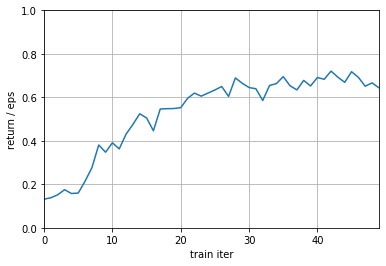Total reward: 0.874
Total length: 7

Total reward: 0.91
Total length: 5

Total reward: 0.856
Total length: 8


## 5. Testing on more complex environments¶

Now that we have seen that we can solve a very simple environment with vanilla policy gradients, let's try harder ones and see how the algorithm behaves!

We start with a bigger gridworld. This makes the problem sparser, i.e. the algorithm sees less rewards early on in training.

In :
# hyperparameters
policy_params = ParamDict(
policy_class = Policy,    # Policy class to use (replaced later)
hidden_dim = 32,          # dimension of the hidden state in actor network
learning_rate = 1e-3,     # learning rate of policy update
batch_size = 1024,        # batch size for policy update
policy_epochs = 4,        # number of epochs per policy update
entropy_coef = 0.001,     # hyperparameter to vary the contribution of entropy loss
)
params = ParamDict(
policy_params = policy_params,
rollout_size = 2050,      # number of collected rollout steps per policy update
num_updates = 50,         # number of training policy iterations
discount = 0.99,          # discount factor
plotting_iters = 10,      # interval for logging graphs and policy rollouts
env_name = 'MiniGrid-Empty-8x8-v0',  # we are using a tiny environment here for testing
)

env, rollouts, policy = instantiate(params)
rewards, success_rate = train(env, rollouts, policy, params)
print("Training completed!")

Training completed!


### Evaluation - bigger gridworld¶

Now again plot the final training curve as well as some policy rollouts.

HINT: The average episode reward should also reach ~0.5 after ~60 iterations. If you can't achieve this result try rerunning your training, randomness can play a big role in the beginning of training.

In :
# final reward + policy plotting for easier evaluation
for _ in range(3):
log_policy_rollout(policy, params.env_name, pytorch_policy=True)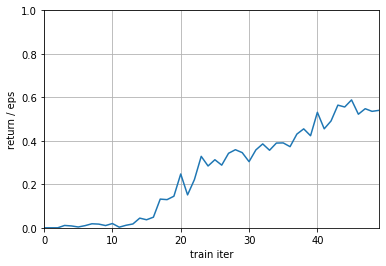Total reward: 0.802
Total length: 11

Total reward: 0
Total length: 50

Total reward: 0.6399999999999999
Total length: 20


##### Comparing the training curves for the two grids of different size...¶

the actor in the smaller gridworld achieved greater average episode rewards in fewer iterations (~15 iterations vs ~40 iterations to achieve avg rewards >0.5). Moreover, in the larger gridworld, the first 10 iterations showed little to no learning while the agent in the smaller gridworld started improving within the first few iterations.

##### The bigger maze tends to learn slower in the beginning of training...¶

because the difference in expected reward values for different actions is small when the distance between the goal and the actor is large. Moreover, the larger the distance, the more possible paths there are to explore.

## 6. Exploring the effect of Reward Design¶

Next we will test our algorithm on an even more challenging environment that includes obstacles in the form of walls and a bottleneck doorway the agent needs to pass through to reach the goal.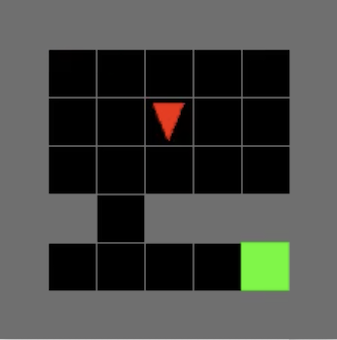In this environment we will investigate the effect of different reward choices on the training dynamics and later how we can improve the vanilla REINFORCE algorithm we implemented above.

Rewards are central to RL as they provide guidance to the policy about the desired agent behavior. There are many different ways to specify rewards and each of them has their own trade-offs: from easy to specify but hard to learn all the way to challenging to specify, but easy learning. Let's explore the trade-offs we can make!

### Experimentation with multiple seeds¶

Before we move on to experimentation, an important aspect in reinforcement learning is to understand the impact of seed variability on experiments. Different seeds can affect training and exploration a lot, therefore it is common practice in RL research to run multiple seeds and plot the mean $\pm$ std of the results. This problem becomes more adverse on more complex environments, as randomness can have bigger influence on the results of training.

Therefore, for the following experiments, we will follow this practice and use three random seeds

In :
n_seeds = 3


Try running the previous settings (bigger gridworld environment) with 3 seeds:

In :
rewards, success_rates = [], []
for i in range(n_seeds):
print("Start training run {}!".format(i))
env, rollouts, policy = instantiate(params)
r, sr = train(env, rollouts, policy, params, seed=i)
rewards.append(r); success_rates.append(sr)
print('All training runs completed!')


All training runs completed!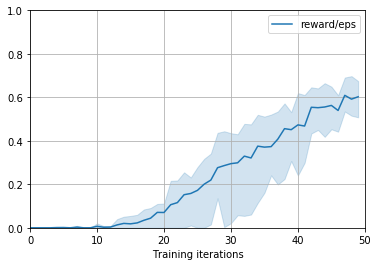### Sparse Reward Environment¶

We start with the easiest to define version of rewards: sparse rewards. The idea is that we just define a very sparse signal, usually an indicator that some goal was achieved. This is in most cases pretty convenient, e.g. we can just detect whether our agent reached the goal and provide a positive reward if that was the case.

The limitations of this formulation become quickly apparent when we think of more complex tasks. For example if an autonomous car would only receive a reward once it drove us to our goal location it would not get a lot of guidance on the way. As a rule of thumb: sparse rewards are easy to specify but usually hard to learn from.

We explore sparse rewards in our test environment by providing a reward of one when the agent reaches the target (minus a small penalty depending on how long it took the agent to get there) and zero reward otherwise. Run the training code on this new environment and see how the algorithm behaves. Since these environments are harder, we train it for longer (see num_updates).

In :
# Load the environment
from minigrid_utils import DeterministicCrossingEnv
import warnings

def gen_wrapped_env(env_name):
if env_name is 'det_sparse':
warnings.filterwarnings("ignore")   # suppress warning when plotting
return wrap_env(FlatObsWrapper(DeterministicCrossingEnv()))
return wrap_env(FlatObsWrapper(gym.make(env_name)))

In :
# hyperparameters
policy_params = ParamDict(
policy_class = Policy,    # Policy class to use (replaced later)
hidden_dim = 32,          # dimension of the hidden state in actor network
learning_rate = 1e-3,     # learning rate of policy update
batch_size = 1024,        # batch size for policy update
policy_epochs = 4,        # number of epochs per policy update
entropy_coef = 0.001,     # hyperparameter to vary the contribution of entropy loss
)
params = ParamDict(
policy_params = policy_params,
rollout_size = 2050,      # number of collected rollout steps per policy update
num_updates = 200,        # number of training policy iterations
discount = 0.99,          # discount factor
plotting_iters = 10,      # interval for logging graphs and policy rollouts
env_name = 'det_sparse',  # we are using a tiny environment here for testing
)

rewards_sparse, success_rates_sparse = [], []
for i in range(n_seeds):
print("Start training run {}!".format(i))
env, rollouts, policy = instantiate(params, nonwrapped_env=DeterministicCrossingEnv())
r, sr = train(env, rollouts, policy, params, seed=i)
rewards_sparse.append(r); success_rates_sparse.append(sr)
print('All training runs completed!')

All training runs completed!

In :
plot_learning_curve(rewards_sparse, success_rates_sparse, params.num_updates, plot_std=True)
for _ in range(3):
log_policy_rollout(policy, params.env_name, pytorch_policy=True)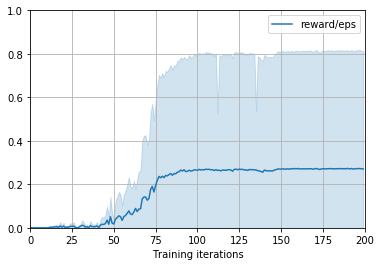Total reward: 0
Total length: 50

Total reward: 0
Total length: 50

Total reward: 0
Total length: 50


#### "Densify" Reward¶

Sparse rewards are easy to specify but only provide very little feedback to the algorithm for what constitutes desirable behavior. As you have seen in the previous examples, this makes it very challenging to effectively learn a good policy. In practice, we therefore usually resort to constructing a more dense reward that gives the policy more "guidance" during learning. How to make the reward more dense is highly problem-dependent.

Below we will test two custom environments that provide different options for a more dense reward on the obstacle-maze task and investigate whether this helps training the policy.

First we will overwrite the gen_wrapped_env(...) method used for logging such that it can handle the new environments.

In :
from minigrid_utils import (DeterministicCrossingEnv, DetHardEuclidCrossingEnv,
DetHardSubgoalCrossingEnv)
import warnings

def gen_wrapped_env(env_name):
if env_name is 'det_sparse':
warnings.filterwarnings("ignore")   # suppress warning when plotting
return wrap_env(FlatObsWrapper(DetSparseCrossingEnv()))
if env_name is 'det_subgoal':
warnings.filterwarnings("ignore")   # suppress warning when plotting
return wrap_env(FlatObsWrapper(DetHardSubgoalCrossingEnv()))
elif env_name is 'det_euclid':
warnings.filterwarnings("ignore")   # suppress warning when plotting
return wrap_env(FlatObsWrapper(DetHardEuclidCrossingEnv()))
return wrap_env(FlatObsWrapper(gym.make(env_name)))


### Subgoal Reward¶

We will first try a hybrid solution between a sparse setup where the agent receives rewards only when the goal is reached and a truly dense setup where the agent receives reward in every step. Concretely, we will add one more subgoal to the environment to encourage the agent to reach the bottleneck state that passes through the wall. Upon reaching the subgoal the agent will receive a positive reward, encouraging it to reach the bottleneck more often. Laying out such a "path of breadcrumbs" is a standard practice in RL and we will explore whether our agent can benefit from it.

Execute the cells below to train the algorithm on the subgoal-based environment.

In :
# hyperparameters
policy_params = ParamDict(
policy_class = Policy,    # Policy class to use (replaced later)
hidden_dim = 32,          # dimension of the hidden state in actor network
learning_rate = 1e-3,     # learning rate of policy update
batch_size = 1024,        # batch size for policy update
policy_epochs = 4,        # number of epochs per policy update
entropy_coef = 0.001,     # hyperparameter to vary the contribution of entropy loss
)
params = ParamDict(
policy_params = policy_params,
rollout_size = 2050,      # number of collected rollout steps per policy update
num_updates = 200,        # number of training policy iterations
discount = 0.99,          # discount factor
plotting_iters = 10,      # interval for logging graphs and policy rollouts
env_name = 'det_subgoal',  # we are using a tiny environment here for testing
)

rewards_subgoal, success_rates_subgoal = [], []
for i in range(n_seeds):
print("Start training run {}!".format(i))
env, rollouts, policy = instantiate(params, nonwrapped_env=DetHardSubgoalCrossingEnv())
r, sr = train(env, rollouts, policy, params, seed=i)
rewards_subgoal.append(r); success_rates_subgoal.append(sr)
print('All training runs completed!')

All training runs completed!

In :
plot_learning_curve(rewards_subgoal, success_rates_subgoal, params.num_updates, plot_std=True)
for _ in range(3):
log_policy_rollout(policy, params.env_name, pytorch_policy=True)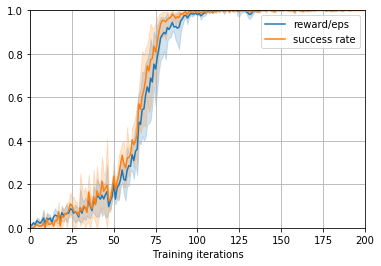Total reward: 1.02
Total length: 10

Total reward: 1.02
Total length: 10

Total reward: 1.02
Total length: 10


### Dense Reward (distance-based)¶

While adding subgoals provided some guidance signal to the agent, we will try to further "densify" the reward formulation. Concretely, we will explore a rather straightforward way to define a dense reward: we will use the euclidean distance $d$ between the current agent position to the goal to define a reward.

At this point one could be tempted to simply use the complement $1-d$ as reward such that maximizing it would lead the agent to minimize the distance to the goal. This is a good example for why specifying dense rewards is hard! If we were to choose this simple reward formulation the agent would actually be encouraged to move next to the goal, but then wait next to the goal until right before the timeout of the episode is reached as terminating early is worse than receiving a long stream of high rewards while waiting next to the goal.

To prevent such "lazyness", we instead look at the differnce of the current distance to the goal and the previous step's distance. If this difference is negative, i.e. if we moved closer to the goal with the last action, we reward the agent. If we moved away from the goal we instead return a negative reward to the agent. If we distance does not change, i.e. if the agent stays in place, we do not provide any reward. In this way the agent is encouraged to choose actions that bring it closer to the goal while there is no benefit to waiting next to the goal (the discount factor <1 ensures that waiting is actually a bad choice).

Run the following cells to train an agent on the euclidean-distance-based dense reward environment.

In :
# hyperparameters
policy_params = ParamDict(
policy_class = Policy,    # Policy class to use (replaced later)
hidden_dim = 32,          # dimension of the hidden state in actor network
learning_rate = 1e-3,     # learning rate of policy update
batch_size = 1024,        # batch size for policy update
policy_epochs = 4,        # number of epochs per policy update
entropy_coef = 0.001,     # hyperparameter to vary the contribution of entropy loss
)
params = ParamDict(
policy_params = policy_params,
rollout_size = 2050,      # number of collected rollout steps per policy update
num_updates = 200,        # number of training policy iterations
discount = 0.99,          # discount factor
plotting_iters = 10,      # interval for logging graphs and policy rollouts
env_name = 'det_euclid',  # we are using a tiny environment here for testing
)

rewards_dense, success_rates_dense = [], []
for i in range(n_seeds):
print("Start training run {}!".format(i))
env, rollouts, policy = instantiate(params, nonwrapped_env=DetHardEuclidCrossingEnv())
r, sr = train(env, rollouts, policy, params, seed=i)
rewards_dense.append(r); success_rates_dense.append(sr)
print('All training runs completed!')

All training runs completed!

In :
plot_learning_curve(rewards_dense, success_rates_dense, params.num_updates, plot_std=True)
for _ in range(3):
log_policy_rollout(policy, params.env_name, pytorch_policy=True)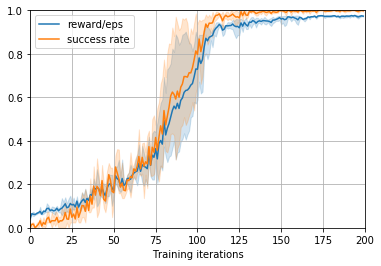Total reward: 0.98
Total length: 10

Total reward: 0.98
Total length: 10

Total reward: 0.872
Total length: 16


### Optimal Dense Reward¶

In the previous example we saw that adding dense rewards can help speeding up training, however there were some degeneracies which discouraged the agent from learning the optimal policy. This process is typical when tackling a new task with RL: one tries sparse rewards and then keeps increasing the density of the reward until the agent is able to learn the desired behavior. Along the way the agent will discover various ways in which to exploit the different reward choices (remember the "lazy" behavior described above), requiring tedious changes to the reward design. This process, known as "reward engineering" is a big pain when developing RL solutions.

In the following we will sidestep this process. In our simple obstacle maze environment it is relatively straightforward to define a reward that is likely very close to "optimal". We will alter the dense reward from the previous section to use the shortest-path distance between the agent and the goal. We do this by computing the manhattan distance while taking the walls into account.

In the following code, implement manhattan distance, and then run your custom environment below.

In :
from minigrid_utils import DetHardOptRewardCrossingEnv

class CustomEnv(DetHardOptRewardCrossingEnv):
def manhattan_distance(self, x, y):
distance = np.sum(np.abs(x-y))
return distance

def gen_wrapped_env(env_name):
if env_name is 'det_sparse':
warnings.filterwarnings("ignore")   # suppress warning when plotting
return wrap_env(FlatObsWrapper(DetSparseCrossingEnv()))
elif env_name is 'det_subgoal':
warnings.filterwarnings("ignore")   # suppress warning when plotting
return wrap_env(FlatObsWrapper(DetHardSubgoalCrossingEnv()))
elif env_name is 'det_euclid':
warnings.filterwarnings("ignore")   # suppress warning when plotting
return wrap_env(FlatObsWrapper(DetHardEuclidCrossingEnv()))
elif env_name is 'custom_env':
warnings.filterwarnings("ignore")   # suppress warning when plotting
return wrap_env(FlatObsWrapper(CustomEnv()))
return wrap_env(FlatObsWrapper(gym.make(env_name)))

In :
# hyperparameters
policy_params = ParamDict(
policy_class = Policy,    # Policy class to use (replaced later)
hidden_dim = 32,          # dimension of the hidden state in actor network
learning_rate = 1e-3,     # learning rate of policy update
batch_size = 1024,        # batch size for policy update
policy_epochs = 4,        # number of epochs per policy update
entropy_coef = 0.001,     # hyperparameter to vary the contribution of entropy loss
)
params = ParamDict(
policy_params = policy_params,
rollout_size = 2050,      # number of collected rollout steps per policy update
num_updates = 200,        # number of training policy iterations
discount = 0.99,          # discount factor
plotting_iters = 10,      # interval for logging graphs and policy rollouts
env_name = 'custom_env',  # we are using a tiny environment here for testing
)

rewards_custom, success_rates_custom = [], []
for i in range(n_seeds):
print("Start training run {}!".format(i))
env, rollouts, policy = instantiate(params, nonwrapped_env=CustomEnv())
r, sr = train(env, rollouts, policy, params, seed=i)
rewards_custom.append(r); success_rates_custom.append(sr)
print('All training runs completed!')

All training runs completed!

In :
plot_learning_curve(rewards_custom, success_rates_custom, params.num_updates, plot_std=True)
for _ in range(3):
log_policy_rollout(policy, params.env_name, pytorch_policy=True)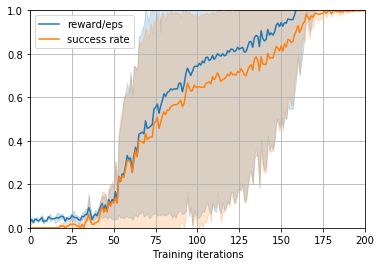Total reward: 1.17
Total length: 10

Total reward: 1.17
Total length: 10

Total reward: 1.17
Total length: 10


### Compile Reward Design Results¶

In order to make comparison across reward design experiments easier, please run the following code block to make a combined code block.

In :
from utils import plot_grid_std_learning_curves
plots = {
'Sparse': (rewards_sparse, success_rates_sparse),
'Subgoal': (rewards_subgoal, success_rates_subgoal),
'Dense': (rewards_dense, success_rates_dense),
'Custom': (rewards_custom, success_rates_custom)}

plt.rcParams['figure.figsize'] = (18.0, 14.0) # set default size of plots
plt.rcParams['figure.figsize'] = (10.0, 8.0) # set default size of plots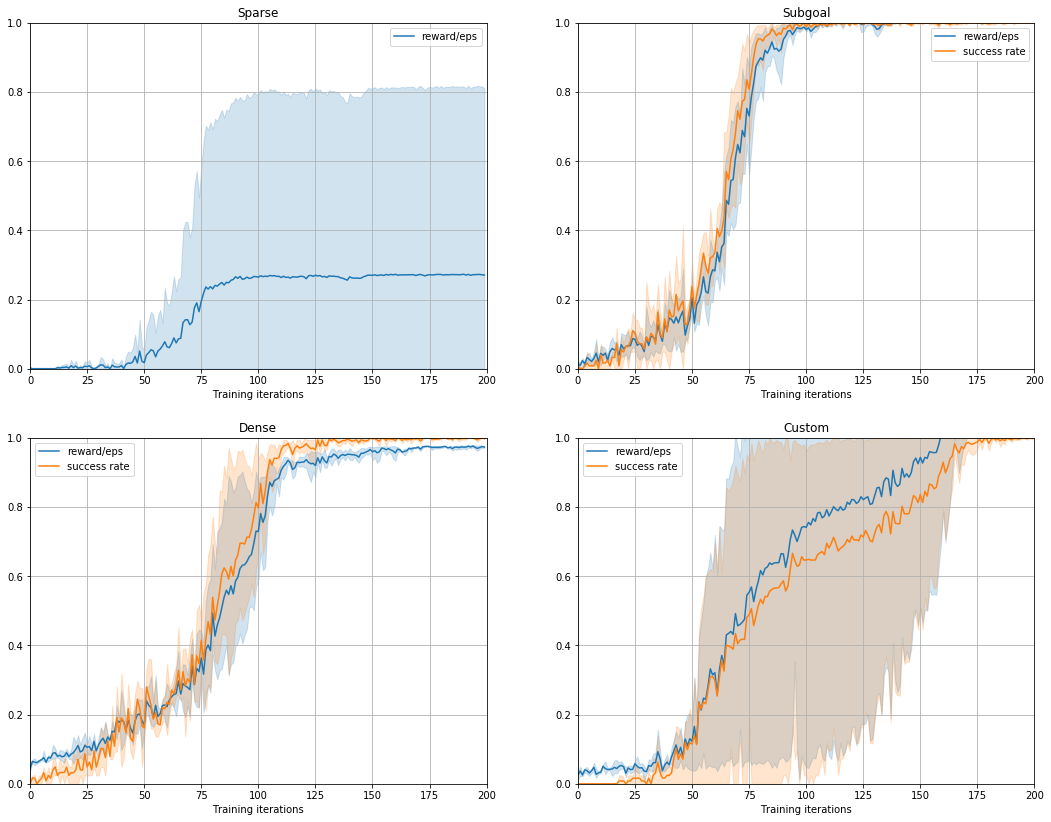Comparing the performance of the different dense reward formulations... While the custom formulation achieved the highest average reward after 200 iterations (1.17), it also had the greatest variation between seeds and the greatest difference between average reward and success rate. While dense and subgoal learning appeared to optimize success rate, the custom formulation optimized reward over success. Because subgoal formulation had least variability and steepest/fastest improvement, it is the best for the given gridworld.

Dense rewards in the real world... It is easy to design dense rewards for simple tasks that have clear milestones in known/deterministic environments (ex. teaching a dog to roll over by giving it treats as it progressively learns to sit, laydown, and finally roll over). It is not possible for applications in non-deterministics state spaces such as in autonomous driving/cars.

If a dense reward is not available, changing the distance measure may improve training. If the agent can't move diagonally, it may be useful changing from euclidian distance to manhattan distance so reward evaluations are more accurate. Additionally, adding a penalty for each step taken encourages the agent to learn the shortest/fastest path and discourages laziness.

## 7. Add a Baseline Network¶

Since REINFORCE relies on sampling-based gradient estimates to update the policy, the gradients can be very noisy, which can make the training unstable and slow. There are many ways which attempt to reduce the variance in learning, and one such way is to use a baseline.

The key idea of a baseline is that if we add or subtract a term $b(s_t)$ which is independent of action to the estimated returns $V_t$, then the expected value still remains the same:

$$\nabla J(\theta) = \mathbb{E}_{\pi}\big[ \nabla_{\theta} \log \pi_{\theta}(a, s) \; V_t(s) \big] = \mathbb{E}_{\pi}\big[ \nabla_{\theta} \log \pi_{\theta}(a, s) \; [V_t(s) - b_t(s)] \big]$$

Hence, this keeps the gradient estimates unbiased (that is close to real gradients), and can help in reducing the variance if it is able to reduce the magnitude of gradients. Reduced variance can potentially lead to more stable training.

A good baseline to use is the value function of a state $b_t(s) = v_t(s)$, which can be trained along with the policy. The value function acts like a critic to the actor (policy network), where the critic's objective is to estimate correct expected returns $V_t(s)$ and actor's objective is the policy gradient augmented by critic's predictions.

The term $V_t(s) - v_t(s)$ is called the advantage, and it measures how well the policy is expected to do (estimated by expected rewards) as compared to the average value of a state (estimated by value function).

In :
class CriticNetwork(nn.Module):
def __init__(self, num_inputs, hidden_dim):
super().__init__()

####################################################################################
### 1. Build the Actor network as a torch.nn.Sequential module                   ###
###    with the following layers:                                                ###
###        (1) a Linear layer mapping from input dimension to hidden dimension   ###
###        (2) a Tanh non-linearity                                              ###
###        (3) a Linear layer mapping from hidden dimension to hidden dimension  ###
###        (4) a Tanh non-linearity                                              ###
###        (5) a Linear layer mapping from hidden dimension to appropriate       ###
###            dimension                                                         ###
### Note: We do not need an activation on the output, because the actor is       ###
###       predicting a value, which can be any real number                       ###
####################################################################################
self.fc = nn.Sequential(
nn.Linear(num_inputs, hidden_dim),
nn.Tanh(),
nn.Linear(hidden_dim,hidden_dim),
nn.Tanh(),
nn.Linear(hidden_dim,1),

)

def forward(self, state):
x = self.fc(state)
return x

class ACPolicy(Policy):
def __init__(self, num_inputs, num_actions, hidden_dim, learning_rate, batch_size, policy_epochs,
entropy_coef=0.001, critic_coef=0.5):
super().__init__(num_inputs, num_actions, hidden_dim, learning_rate, batch_size, policy_epochs, entropy_coef)

self.critic = CriticNetwork(num_inputs, hidden_dim)

####################################################################################
### Create a common optimizer for actor and critic with the given learning rate  ###
### (requires 1-line of code)                                                    ###
####################################################################################

self.critic_coef = critic_coef

def update(self, rollouts):
print_vals=0
for epoch in range(self.policy_epochs):
data = rollouts.batch_sampler(self.batch_size)

for sample in data:
actions_batch, returns_batch, obs_batch = sample
log_probs_batch, entropy_batch = self.evaluate_actions(obs_batch, actions_batch)

value_batch = self.critic(obs_batch)

####################################################################################
### 1. Compute the mean loss for the policy update using action log-             ###
### 2. Compute the mean entropy for the policy update                            ###
### 3. Compute the critic loss as MSE loss between estimated value and expected  ###
###     returns.                                                                 ###
####################################################################################
entropy_loss = torch.mean(entropy_batch).mul(-1) #/self.batch_size

critic_loss = F.mse_loss(returns_batch,value_batch)

loss = policy_loss + \
self.critic_coef * critic_loss + \
self.entropy_coef * entropy_loss

loss.backward()
self.optimizer.step()

@property
def num_params(self):
return super().num_params + count_model_params(self.critic)

In :
# hyperparameters
policy_params = ParamDict(
policy_class = ACPolicy,  # Policy class to use (replaced later)
hidden_dim = 32,          # dimension of the hidden state in actor network
learning_rate = 1e-3,     # learning rate of policy update
batch_size = 1024,        # batch size for policy update
policy_epochs = 4,        # number of epochs per policy update
entropy_coef = 0.001,     # hyperparameter to vary the contribution of entropy loss
critic_coef = 0.5         # Coefficient of critic loss when weighted against actor loss
)
params = ParamDict(
policy_params = policy_params,
rollout_size = 2050,      # number of collected rollout steps per policy update
num_updates = 200,        # number of training policy iterations
discount = 0.99,          # discount factor
plotting_iters = 10,      # interval for logging graphs and policy rollouts
env_name = 'custom_env',  # we are using a tiny environment here for testing
)

rewards, success_rates = [], []
for i in range(n_seeds):
print("Start training run {}!".format(i))
env, rollouts, policy = instantiate(params, nonwrapped_env=CustomEnv())
r, sr = train(env, rollouts, policy, params, seed=i)
rewards.append(r); success_rates.append(sr)
print('All training runs completed!')

All training runs completed!

In :
plot_learning_curve(rewards, success_rates, params.num_updates, plot_std=True)
for _ in range(3):
log_policy_rollout(policy, params.env_name, pytorch_policy=True)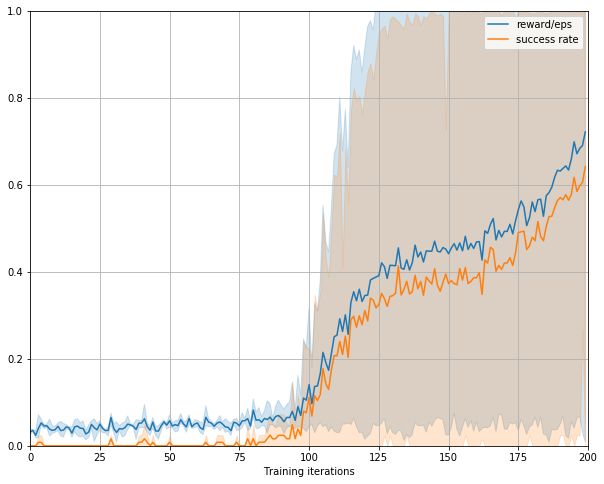Total reward: 1.13
Total length: 15

Total reward: 1.1340000000000001
Total length: 12

Total reward: 1.098
Total length: 14

##### Actor critic algorithm perform vs Vanilla policy gradients¶

The actor critic algorithm has very little learning initially, but then once it begins to show learning, it learns quickly and better (greater av reward at the end of training). The vanilla in comparison has less of a slow start, but also lesser acceleration in learning.

However, with only the graphs above, it is not possible to make absolute generalizations about the formulations. The significant variation in performance between seeds along with the few number of samples puts into question any improvements in average reward due to the algorithm

## 8. Proximal Policy Optimization (PPO)¶

PPO is an actor-critic algorithm which constrains the gradient updates to a policy by modifying the training objective with a clipped surrogate objective. This essentially results in a more stable optimization procedure, and hence leads to better sample efficiency and fast computation time, as compared to vanilla policy gradient methods. It is very easy to implement, and here you are supposed to modify the update function of Actor Critic Policy to incorporate these surrogate losses.

$$ratio = \frac{\pi^{new}(a)}{\pi^{old}(a)} = e^{log \pi^{new}(a) - log \pi^{old}(a)}$$$$\mathcal{L}_{surrogate1} = ratio * Advantage$$$$\mathcal{L}_{surrogate2} = clip (ratio, 1 - c, 1 + c) * Advantage$$$$\mathcal{L}_{policy} = - min(\mathcal{L}_{surrogate1}, \mathcal{L}_{surrogate2})$$
In :
class PPO(ACPolicy):
def update(self, rollouts):
self.clip_param = 0.2
for epoch in range(self.policy_epochs):
data = rollouts.batch_sampler(self.batch_size, get_old_log_probs=True)

for sample in data:
actions_batch, returns_batch, obs_batch, old_log_probs_batch = sample
log_probs_batch, entropy_batch = self.evaluate_actions(obs_batch, actions_batch)

value_batch = self.critic(obs_batch)

old_log_probs_batch = old_log_probs_batch.detach()

ratio = torch.exp(log_probs_batch.sub(old_log_probs_batch))

policy_loss = torch.mean(-torch.min(surr1,surr2))
entropy_loss = torch.mean(entropy_batch).mul(-1)
critic_loss = F.mse_loss(returns_batch,value_batch)

loss = policy_loss + \
self.critic_coef * critic_loss + \
self.entropy_coef * entropy_loss

loss.backward(retain_graph=False)
self.optimizer.step()

In :
# hyperparameters
policy_params = ParamDict(
policy_class = PPO,  # Policy class to use (replaced later)
hidden_dim = 32,          # dimension of the hidden state in actor network
learning_rate = 1e-3,     # learning rate of policy update
batch_size = 1024,        # batch size for policy update
policy_epochs = 4,        # number of epochs per policy update
entropy_coef = 0.001,     # hyperparameter to vary the contribution of entropy loss
critic_coef = 0.5         # Coefficient of critic loss when weighted against actor loss
)
params = ParamDict(
policy_params = policy_params,
rollout_size = 2050,      # number of collected rollout steps per policy update
num_updates = 200,        # number of training policy iterations
discount = 0.99,          # discount factor
plotting_iters = 10,      # interval for logging graphs and policy rollouts
env_name = 'custom_env',  # we are using a tiny environment here for testing
)

rewards, success_rates = [], []
for i in range(n_seeds):
print("Start training run {}!".format(i))
env, rollouts, policy = instantiate(params, nonwrapped_env=CustomEnv())
r, sr = train(env, rollouts, policy, params, seed=i)
rewards.append(r); success_rates.append(sr)
print('All training runs completed!')

All training runs completed!

In :
plot_learning_curve(rewards, success_rates, params.num_updates, plot_std=True)
for _ in range(3):
log_policy_rollout(policy, params.env_name, pytorch_policy=True)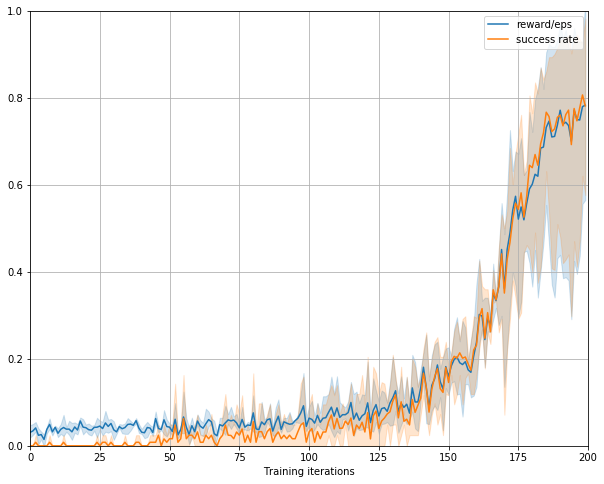Total reward: 0.972
Total length: 21

Total reward: 1.1520000000000001
Total length: 11

Total reward: 0.5399999999999999
Total length: 45

##### PPO vs Actor-critic method¶

There is less variability due to randomness (seed) with PPO, but it also takes longer for the method to begin showing improvements in learning/average reward.

One big problem is choosing a method when the state space is not completely known and variable. As shown from the experiments above, the performance of the method varies significantly between different state spaces. Another problem is defining dense rewards or subgoals, which appears to be the most effective in improving training/learning. Finally, the the increase in training required to learn anything of value grows dramatically as the state space increases.

## Playing with more complex environments!¶

Until this point, we only dealt with static environments, where the walls do not change across episodes. For this last open-ended task, let's add some complexity and use the wall environment (bigger and randomized version)

In :
from minigrid_utils import LavaEnv, WallEnv

def gen_wrapped_env(env_name):
if env_name is 'lava':
warnings.filterwarnings("ignore")   # suppress warning when plotting
return wrap_env(FlatObsWrapper(LavaEnv()))
if env_name is 'wall':
warnings.filterwarnings("ignore")   # suppress warning when plotting
return wrap_env(FlatObsWrapper(WallEnv()))
return wrap_env(FlatObsWrapper(gym.make(env_name)))

In :
#SUBGOAL
# hyperparameters
n_seeds=5
policy_params = ParamDict(
policy_class = Policy,    # Policy class to use (replaced later)
hidden_dim = 32,          # dimension of the hidden state in actor network
learning_rate = 1e-3,     # learning rate of policy update
batch_size = 1024,        # batch size for policy update
policy_epochs = 4,        # number of epochs per policy update
entropy_coef = 0.001,     # hyperparameter to vary the contribution of entropy loss
)
params = ParamDict(
policy_params = policy_params,
rollout_size = 2050,      # number of collected rollout steps per policy update
num_updates = 2000,        # number of training policy iterations
discount = 0.99,          # discount factor
plotting_iters = 100,      # interval for logging graphs and policy rollouts
env_name = 'wall',  # we are using a tiny environment here for testing
)

rewards_subgoal, success_rates_subgoal = [], []
for i in range(n_seeds):
print("Start training run {}!".format(i))
env, rollouts, policy = instantiate(params, nonwrapped_env=WallEnv())
r, sr = train(env, rollouts, policy, params, seed=i)
rewards_subgoal.append(r); success_rates_subgoal.append(sr)
print('All training runs completed!')

# #ACPOLICY
# # hyperparameters
# n_seeds=5;
# policy_params = ParamDict(
#     policy_class = ACPolicy,  # Policy class to use (replaced later)
#     hidden_dim = 32,          # dimension of the hidden state in actor network
#     learning_rate = 1e-4,     # learning rate of policy update
#     batch_size = 1024,        # batch size for policy update
#     policy_epochs = 4,        # number of epochs per policy update
#     entropy_coef = 0.005,     # hyperparameter to vary the contribution of entropy loss
#     critic_coef = 0.75         # Coefficient of critic loss when weighted against actor loss
# )
# params = ParamDict(
#     policy_params = policy_params,
#     rollout_size = 2050,      # number of collected rollout steps per policy update
#     num_updates = 200,        # number of training policy iterations
#     discount = 0.5,          # discount factor
#     plotting_iters = 10,      # interval for logging graphs and policy rollouts
#     env_name =r'wall',
# )
# rewards, success_rates = [], []

# for i in range(n_seeds):
#     print("Start training run {}!".format(i))
#     env, rollouts, policy = instantiate(params,nonwrapped_env=WallEnv())
#     r, sr = train(env, rollouts, policy, params, seed=i)
#     rewards.append(r); success_rates.append(sr)
# print('All training runs completed!')

All training runs completed!

In :
# plot_learning_curve(rewards, success_rates, params.num_updates, plot_std=True)
# for _ in range(n_seeds):
#     log_policy_rollout(policy, params.env_name, pytorch_policy=True)